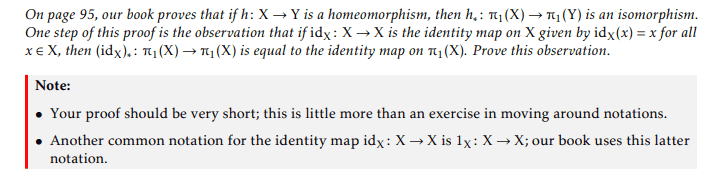# Proof involving Identity maps

• I
Mikaelochi
TL;DR Summary
Another problem from a topology course I took and never really gotSo, this problem I sort of get conceptually but I don't know how I can possibly rewrite (idX)∗ : π1(X) → π1(X). Does this involve group theory? It's supposed to be simple but I honestly I don't see how. Again, any help is greatly appreciated. Thanks.

If $f : X \to Y$ is continuous, then $f_{*} : \pi_1(X) \to \pi_1(Y)$ is defined by $$f_{*}(\gamma) : [0,1] \to Y : t \mapsto (f \circ \gamma)(t)$$ for each $\gamma \in \pi_1(X)$.
If $X = Y$ and $f$ is the identity on $X$, then $f_{*}(\gamma) = \gamma$ and $f_{*}$ is the identity on $\pi_1(X)$.
•